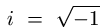# An Algorithm for Forward Fitting

The intent of the Forward-Fitting process is to provide a parametric model which is a best fit to the observed count rate profiles. Ideally, this can be done without making maps of any kind. The idea is to find a model with the fewest parameters for a given c2 or C-statistic giving the "goodness-of-fit" of the computed model modulation profile to the observed profile. The model can be a superposition of circular Gaussians or a superposition of more complicated basis functions.

We restrict ourselves initially to models with two circular Gaussians. Such a model has 8 free parameters:

• (x1,y1),(x2,y2) for the centroids of the sources,
• F1, F2, for their fluxes,
• and w1, w2 for their widths.

It is easy to show that the signal from a single sub-collimator cannot distinguish the difference between a point source and a circular Gaussian, so with little loss of generality, we can start with the case of two point sources. (Later, the algorithm distinguishes between Gaussians and point sources by comparing successive detector profiles.)

Our strategy proceeds as follows:

1. Find the centroid of the source. This can be done in various ways:
• Find the best correlation between the single-revolution signal f(roll_angle) from the coarsest collimator and a function of the form:
f=cos(A*cos(roll_angle-B)-C)
This is very fast and the values of A and B lead to the azimuth and phase of the flare source.
• OR: Make a coarse back-projection map using the two coarsest subcollimators, and find the peak of the map.
2. Make calibrated event lists for all collimators using the flare centroid for map center.
3. Using the profiles of the phase_map_ctr for each collimator, regularize the count rate and make corrections for gridtran, livetime and modamp variations. (Regularization is required for successful interpretation of fourier spectra and for phase shifting.)
( hsi_rebin.pro, hsi_regularize.pro)
4. Compute the power spectrum AS(i)( n), i=1-9 of each of the 9 regularized profiles.
5. Determine the location of significant peaks in the appropriate ranges of each AS(i)( n).
Look for double peaks, starting with the coarsest collimators and working towards the finest. At a given pitch, source separations greater than that pitch will produce a double peak. This will usually be seen in signals from 2 or 3 successive collimators with pitches spanning the separation size. The separation of the sources can then be directly determined from the power-spectral maxima ( n1, n2).
6. For collimators with | n1 - n2| significantly greater than 0. there will be a slow amplitude modulation ("beating") of the signal.
The beat signal is best understood as a long-wavelength waveform in the U-V plane, which modulates the visibilities. (See Figure) The beat waveform, like the parent visibility, has amplitude, phase and direction. The direction of this beat waveform is the same as the direction of the separation vector for the source pair. In general this direction is not readily found directly from the power spectra AS(i) ( n), because the signal has been regularized from the point of view of the flare centroid, which is almost always a different direction than the separation vector, Given the period | n1- n2| and the mean frequency n0 =( n1+ n2)/2 one may find the separation vector by using the Hilbert transform and phase shifting. But first one must filter the spectrum.
• Fourier filter the signal by using only spatial frequencies whose spectral power exceeds 3 s.
• Compute the complex signal fh(k)   =   f(k)   +   i   HILBERT(f),     ()
where HILBERT is a function in the IDL 5.x library.
• Then eliminate the high frequencies by a phase shift:
g(k) = fh(k) X ei n0k
where the shift is given by the peak frequency of the power spectrum.
For two circular Gaussian sources with a radial separation vector, g(k) will be a low-frequency sinusoid.
If the beat amplitude is large (i.e. nearly equal sources), and the separation happens to be radial, the amplitude and phase can be found immediately from g(k). Since in general the separation is nonradial, which causes the the beat signal to be irregularly periodic, it must be found for a complete set of regularizations in different directions:
• Reduce the size of the beat amplitude arrays by rebinning by a factor of 2-4.
• Find the phase of the "beat" signal by re-regularizing g(k) in a range of directions (every 10° or so). The signal with the highest "spectral purity" gives the direction of the separation vector.
( uv_project.pro, spectral_width.pro)
• Alternatively, exploit the fact that the beat amplitude is regularly sampled in one dimension of the UV plane and irregularly sampled in the perpendicular dimension: Compute a 2-D Fourier transform of g(k) using FFT's and direct FT's, and use 2-D power spectra to find the beat amplitude and phase.
• The separation, direction and centroid give x1,x2
7. The total flux on the coarsest scales provides the sum F1+F2.
8. Determine the amplitude of each source distinuished in the Fourier power spectrum.
9. The collimator-to-collimator ratios of the spectral power in each source give the ratios of a Gaussian function of the widths wi and fluxes Fi, from which the final fluxes and widths may be determined. Once the parameters have been found, the model count rate can be compared with the observed one, and the c2 or C-statistic can be computed. If these are sufficiently small, we are done. If not, small adjustments of the parameters can be made in a search for a better fit. If this does not lead to a satisfactory statistic, it will be necessary to seach for a third source or more complicated sources.